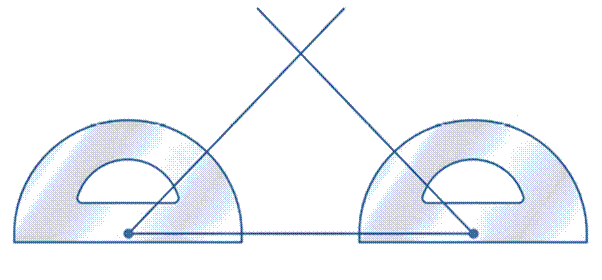Chapter 1.2, Problem 30E### Elementary Geometry for College St...

6th Edition
Daniel C. Alexander + 1 other
ISBN: 9781285195698

#### Solutions

Chapter
Section### Elementary Geometry for College St...

6th Edition
Daniel C. Alexander + 1 other
ISBN: 9781285195698
Textbook Problem
1 views

# On a piece of paper, use your protractor to draw a triangle that has two angles of the same measure. Cut the triangle out of the paper and fold the triangle in half so that the angles of equal measure coincide (one lies over the other). What seems to be true of two of the sides of that triangle?To determine

That what seems to be true of the sides of the triangle which is formed on a piece of paper with two angle of same measure.

Explanation

Calculation:

Given,

When the triangle is folded to a triangle in half so that the two equal angles coincide,

### Still sussing out bartleby?

Check out a sample textbook solution.

See a sample solution

#### The Solution to Your Study Problems

Bartleby provides explanations to thousands of textbook problems written by our experts, many with advanced degrees!

Get Started